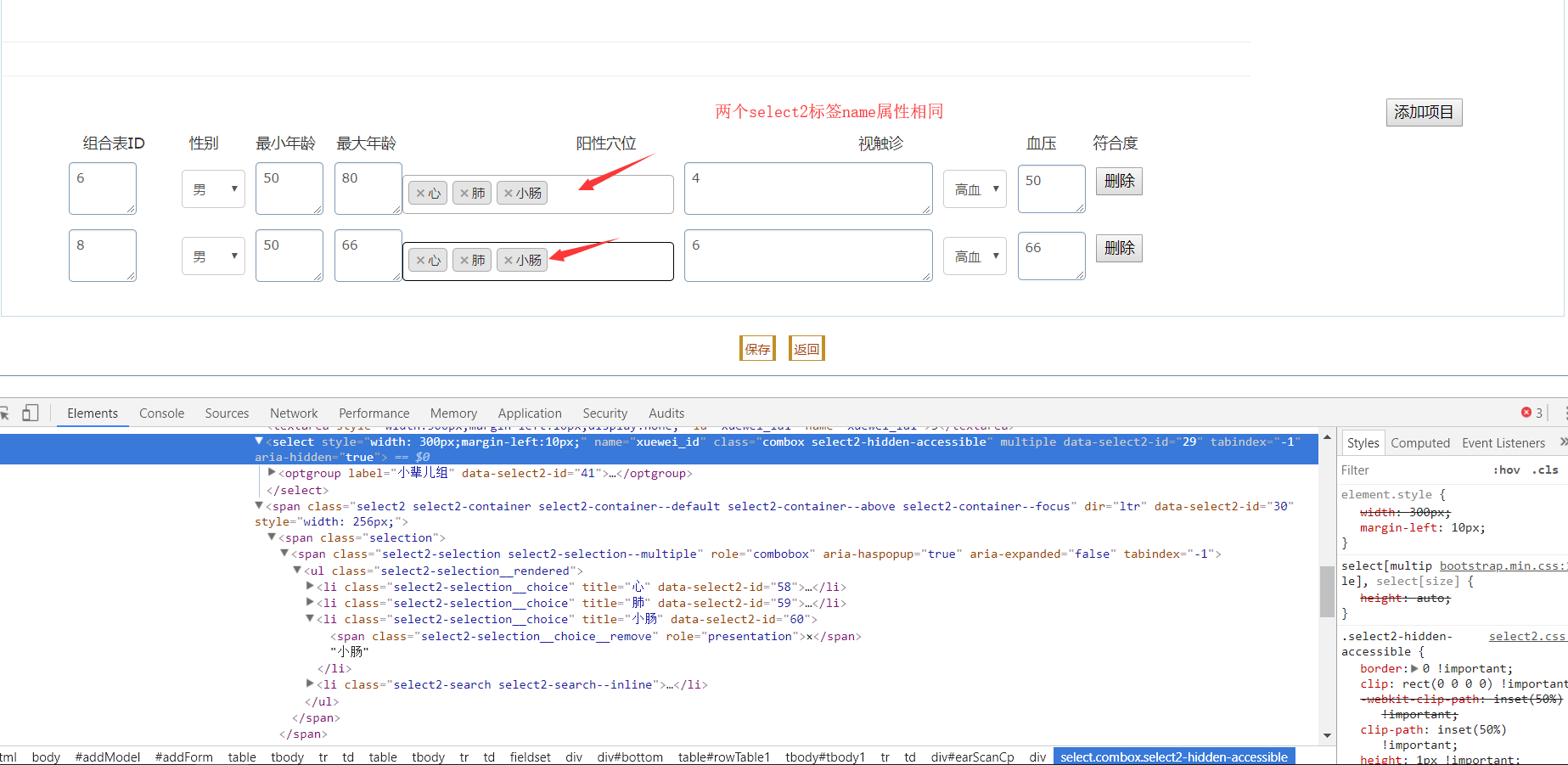name属性相同的两个个select2标签值合并到一个数组里了 如何获取每一个name对应标签的值 20Cjs代码

`````` // 显示修改关键字症状界面
function updateView(id, system_id) {
\$("#btntype").val(1);

\$("#Submit1").click(function() {

});

\$
.ajax({
type : "POST",
url : "/erzhentang/manage/forAjax.do?requestType=12.1",
data : "id=" + id + "&system_id=" + system_id, // 发送到服务器的数据默认id是结论id
success : function(msg) {
\$("#xuewei_id").val("1,2,3").select2();
var json = eval('(' + msg + ')');
document.getElementById("id1").value = json.earScanJielun.id;
document.getElementById("system_id1").value = json.earScanJielun.system_id;
/* 遍历所属系统下所有 耳穴频扫疾病项 */
var arrESJ = eval(json.listEarScanJibing);// arrESJ是一个数组
var checkESJ = document.getElementById("jibing_num1");
var str2 = "";
for (var j = 0; j < arrESJ.length; j++) {
str2 += "<option value=\"" + arrESJ[j].jibing_num
+ "\">" + arrESJ[j].name + "</option>";
var checkESJ2 = arrESJ[j].name;
if (arrESJ[j].jibing_num == json.earScanJielun.jibing_num) {// 疾病编号人为控制每个编号都是唯一
arrESJ[j].name = "selected='selected'";
}
\$("#jibing_num1").html(str2);
}
document.getElementById("jibing_num1").value = json.earScanJielun.jibing_num;
document.getElementById("jielun_rank1").value = json.earScanJielun.jielun_rank;
document.getElementById("jielun1").value = json.earScanJielun.jielun;
/* 下半截部分组合表动态展示修改和添加 */
var arrESC = eval(json.listEarScanCp);// arrESC是一个数组频扫结论组合表
var str = "";
var str1 = "";
for (var i = 0; i < arrESC.length; i++) {
str += "<tr>";
str += "<td colspan=\"8\">";
str += "<div style=\"margin-left: 148px;\" id=\"earScanCp\">";
str += "<div style=\"float: left;\">";
str += "<textarea style=\"width: 50px;margin-left: -86px;position: absolute;\" id=\"cp_id\" name=\"cp_id\" >"
+ arrESC[i].id + "</textarea>";
str += "<select style=\"width: 60px;height: 36px;margin-left: 20px;\" id=\"gender\" name=\"gender\" >"
var myselect = document.getElementById("gender");// 这个获取的是静态jsp页面里标签
/*
* 下拉框主要是应展示项和所有下拉选项的值判断是否对应 分清主和客
* 所有展示项目为主表客表的某一项值和遍历主表的值对应 这里面 下拉框所有选项值是主表
*/
var str1 = "";
for (var j = 0; j < myselect.options.length; j++) {// 下拉框是主家从静态页面获取并且遍历它
str += "<option value=\""
+ myselect.options[j].value
+ "\" "
+ (myselect.options[j].value == arrESC[i].gender ? "selected='selected'"
: "") + ">"
+ myselect.options[j].text + "</option>";
}
"</select>";
str += "<textarea style=\"width: 50px;margin-left: 10px;\" id=\"min_age\" name=\"min_age\" >"
+ arrESC[i].min_age + "</textarea>";
str += "<textarea style=\"width: 50px;margin-left: 10px;\" id=\"max_age\" name=\"max_age\" >"
+ arrESC[i].max_age + "</textarea>";
// str += "<textarea style=\"width:
// 300px;margin-left:10px;\" id=\"xuewei_id\"
// name=\"xuewei_id\" >"
// + arrESC[i].xuewei_id + "</textarea>";
// str +="<div id=\"selectSection\">";
/*
* id=\"xuewei_id\"
*/
str += "<textarea  style=\"width:300px;margin-left:10px;display:none;\" id=\"xuewei_id1\" name=\"xuewei_id1\" >"
+ arrESC[i].xuewei_id + "</textarea>";
//                      str += "<div id=\"selectSection\" name=\"selectSection\">";
str += "<select style=\"width: 300px;margin-left:10px;\"  name=\"xuewei_id\"class=\"combox\" multiple >"
str += "<optgroup label=\"小辈儿组\">";
str += "<option value=\"1\" " + "selected='selected'"
+ ">心</option>";
str += "<option value=\"2\" " + "selected='selected'"
+ ">" + "肺" + "</option>";
str += "<option value=\"3\" " + "selected='selected'"
+ ">" + "小肠" + "</option>";
str += "<option value=\"4\">" + "口" + "</option>";
str += "<option value=\"5\">" + "食道" + "</option>";
str += "</optgroup>";
// str += "</div>";
/* 进一步转换频扫组合表穴位id字符串转array数组 */

/*
* var strXueweiId = arrESC[i].xuewei_id; var
* arrayXueweiId = strXueweiId.split(",");
*
* for (var j = 0; j < arrayXueweiId.length; j++) { str2 += "<option
* value=\"" + arrayXueweiId[j].value + "\">" +
* arrayXueweiId[j].value + "</option>"; var checkESJ2 =
* arrayXueweiId[j].name; if (arrayXueweiId[j] == 5) {
* arrayXueweiId[j] = "selected='selected'"; }
* \$("#jibing_num1").html(str2); }
*/
"</select>";
//
// \$("#xuewei_id").val("1,2,3").select2();

str += "<textarea style=\"width: 220px;margin-left: 10px;\" id=\"chuzhen_id\" name=\"chuzhen_id\" >"
+ arrESC[i].chuzhen_id + "</textarea>";
str += "<select style=\"width: 60px;height: 36px;margin-left: 10px;\" id=\"bp\" name=\"bp\" >"
var myselect1 = document.getElementById("bp");// 这个获取的是静态jsp页面里标签
for (var k = 0; k < myselect1.options.length; k++) {// 下拉框是主家从静态页面获取并且遍历它
str += "<option value=\""
+ myselect1.options[k].value
+ "\" "
+ (myselect1.options[k].value == arrESC[i].bp ? "selected='selected'"
: "") + ">"
+ myselect1.options[k].text + "</option>";
}
"</select>";
str += "<textarea style=\"width: 50px; height: 36px;margin-left: 10px;margin-top: 0px;\" id=\"jielun_conform\" name=\"jielun_conform\">"
+ arrESC[i].jielun_conform + "</textarea>";
str += "</div>";
str += "<div >";
str += " <input type=\"button\" onclick=\"delRow(this,"
+ arrESC[i].id
+ ","
+ arrESC[i].bianzhengId
+ ")\" value=\"删除\" id=\"deleteESC\" name=\"deleteESC\" style=\"float: left; margin-left:10px; margin-top: 5px;\">";
str += "</div>";
str += "</div>";
str += "</td>";
str += "</tr>";
/* 替换静态页面 */
\$("#tbody1").html(str);

select2Execute();
}

}
});
}
``````

`````` /* 执行select2方法 */
function select2Execute() {

\$(function() {

\$("select[name='xuewei_id']").select2({
// \$('select[id="xuewei_id"]').select2({
// \$('#xuewei_id').select2({
// \$("#selectSection").find("select.combox").select2({
// \$(".select2-selection__choice").select2();
// \$(".combox").select2({

placeholder : "请至少选择一个人名",
tags : true,
createTag : function(decorated, params) {
return null;
},
width : '256px'
});

function formatState(state) {
if (!state.id) {
return state.text;
}
var \$state = \$('<span>' + state.text + '</span>');
return \$state;
}
;

\$('#sel_recommender').select2({
placeholder : "请选择一个人名",
templateResult : formatState,
width : '256px'
});

// \$("#selectSection").find("select.xuewei_id").val();
// document.getElementById("selectSection").value=\$("select[name='xuewei_id']").select2('data');
\$("#xuewei_id1").val(
\$("select[name='xuewei_id']").select2('data'));//赋值给textarea隐藏的标签
});
}
``````
0

4个回答

011 个月之前 回复
``````    <script>
\$(function(){
var m1 = \$('select[name="mm"]').value;
var m2 = \$('select[name="mm"]').value;
console.log(m1,m2);
})
</script>

<select name="mm">
<option>1</option>
<option>2</option>
<option>3</option>
<option>4</option>
</select>
<select name="mm">
<option>5</option>
<option>6</option>
<option>7</option>
<option>8</option>
</select>

按这个方法试试  因为有两个 js  查出来 会组成一个数组   在数组中去找对应的  两个select   代表第一个  代表第二个

希望 能帮助到你
``````
0
\$(function(){ var m1 = \$("select[name-re='1']").val(); var m2 = \$("select[name-re='2']").val(); console.log(m1,m2); })
``````            <select name-re="1" name="mm">
<option>1</option>
<option>2</option>
<option>3</option>
<option>4</option>
</select>
<select name-re="2"  name="mm">
<option>5</option>
<option>6</option>
<option>7</option>
<option>8</option>
</select>
``````
0
`````` \$(function(){ var m1 = \$("select[name-re='1']").val(); var m2 = \$("select[name-re='2']").val(); console.log(m1,m2); })
<select name-re="1" name="mm">
<option>1</option>
<option>2</option>
<option>3</option>
<option>4</option>
</select>
<select name-re="2"  name="mm">
<option>5</option>
<option>6</option>
<option>7</option>
<option>8</option>
</select>
``````
0list中对相同name的内容，对count值加和，最终相同name合并成一条数据
package until; /** * Created by csucoderlee on 2019/4/30 * &lt;p&gt; * list中对相同name的内容，对count值加和，最终相同name合并成一条数据 */ import java.util.ArrayList; import java.util.HashMap; import java.util.List; im...
[前台]---js获取input标签中name相同的各个value值

Django表单提交后，如何获取相同name的不同value值
i前端：nput_test.html Search {{ result }} {% csrf_token %} 两个标签name都是'key' 后台：Django views.py def setting(request): if re
js获取相同name的元素个数和获取其中任意一个元素的值（获取最后一个元素值）
html的<meta>标签使用方法，一个name属性，一个content属性，其实就是一个键值对，有键有值
html的标签使用方法，一个name属性，一个content属性，其实就是一个键值对，有键有值
jQuery对所有name为一个值或约等于一个值的标签统一赋值
JSP js: \$("label[name^='year']").each(function(){ \$(this).text("2012"); }); JSP JS: \$("input[name='joby']").each(function (i,n){ \$(this).attr('value',data.list[i]); });
js 实现获取name 相同的页面元素并循环遍历
js 实现获取name 相同的页面元素并循环遍历： \$("input[name='blues']").each(function(j,item){       // 你要实现的业务逻辑      console.log(item.value);  //输出input 中的 value 值到控制台 });
Struts中实体类的字段名称要对应jsp中textfield中name属性值

Servlet 读取 HTML 中同一 NAME 属性值的多个参数

js-file标签的name属性赋值

\$("input:text").attr("name"); \$("input:text").prop("name");  // 也可以使用prop()方法获取属性

jQuery ajax - serialize() 方法 使用js获取表单中各个name的值，不需要一个一个获取了。 http://www.w3school.com.cn/jquery/ajax_serialize.asp
jquery获取相同类标签的所有值

JQ 获取多个相同name 的 input框的 value 值
var img_url =[]; \$(&quot;input[name='goods_images_a']&quot;).each(function(){ img_url.push(\$(this).val()); })
js通过Class Name获取一个对象的数组
DOM 提供了一个名为 getElementById() 的方法，这个方法将返回一个对象，这个对象就是参数 id 所对应的元素节点。另外，getElementByTagName() 方法会返回一个对象的数组，每一个对象分别对应着文档里有给定标签的一个元素。这个方法的参数是 html 标签的名字。现在我们考虑一个问题，能不能通过标签的类名class name来获取该对象呢？下面是这个猜想的程序实

meta 标签之NAME属性 详解
jquery获取html中所有的id值/name值/自定义值----相同的dom元素
-
Struts2的数据标签获取不到Person的name属性解决方案

form表单提交多个下拉框获取select选项值，name相同

-

HTML中标签的属性ID与Name的区别！
ID和Name都可以用来标识一个标记，Javascript分别有两个方法getElementById和getElementByName来定位Dom节点。     区别如下：    1、我们知道在网页做Post提交时，是以Form（即表单域）为单位进行提交的，一个Form里有若干个表单对象(如)，同一个页面里可以为多个Form（Asp.net不同，它只允许有一个，且名字必须为Form1），在表单

table中tr的name属性无法通过getElementsByName获取tr元素

jQuery通过input标签的name获取值
jquery根据name属性查找 \$("div[id]") 选择所有含有id属性的div元素 \$("input[name='keleyicom']") 选择所有的name属性等于'keleyicom'的input元素 \$("input[name!='keleyicom']") 选择所有的name属性不等于'keleyicom'的input元素 \$("input[name^='keleyi']")
checkbox向后台传多个name相同的value时的两种属性驱动接收方式

spring配置文件bean标签 name属性取值语法问题，（新手问题多）--小问题总结都在这里
<beans xmlns:xsi="http://www.w3.org/2001/XMLSchema-instance" xmlns:p="http://www.springframework.org/schema/p" xmlns="http://www.springframework.org/schema/beans" xsi:schemaLocation="http://www.
php获取表单中多个同名元素的值

dom4j获取xml中所有name元素的值（第一个、第二个）
p1.xml
jQuery如何获取同一个类标签的所有的值

django request 获取多个相同name的value值

js获取多个相同name值的input 当前对象